Courses
Courses for Kids
Free study material
Free LIVE classes
More

# Graphical Analysis of Kinematics - JEE Important Topic## What is Kinematics?

Last updated date: 17th Mar 2023
Total views: 77.4k
Views today: 1.56k

Kinematics is a branch of mechanics which is used in studying the motion of a body without considering the cause of the motion. This field of science discusses the motion of the body based on the change in the position of the body in a given interval of time. The topics which are to analyse the motion of the body are distance, displacement, speed, velocity and acceleration.

The motion of the body can be determined by two methods i.e. analytical method and the graphical method. In the analytical method, the motion of the body is evaluated in the form of a mathematical equation with the help of calculus. In the graphical method, the motion of the body is studied based on a graphical representation of the curve of the motion. Kinematics can be studied in all three dimensions.

## Graphical Representation of the Motion of the Body

For a better understanding of the motion of the body, there are three major graphical relationships present in kinematics. These graphical relationships are shown below:

• Displacement-time graphs

• Velocity-time graphs

• Acceleration graphs

In displacement-time graphs, a graph is plotted between the displacement and time to represent the motion of the body in terms of change in position of the body, with respect to the time. On the graph, the displacement is taken on the y-axis and time is on the x-axis.

In velocity-time graphs, a graph is plotted between the velocity and time to represent the motion of a body in terms of the change in velocity of the body, with respect to the time. While plotting the graph, the velocity is taken on the y-axis and time is on the X-axis.

In acceleration-time graphs, a graph is plotted between the acceleration and time to represent the motion of the body in terms of change in acceleration of the body, with respect to time. The acceleration is taken on the y-axis and time is on the x-axis.

## Kinematics Graphs

### 1. When the Body is Stationary:

The displacement-time graphical representation represents that the change in the position of the body with respect to time is zero. The graph for a stationary body is shown by a straight line parallel to the x-axis.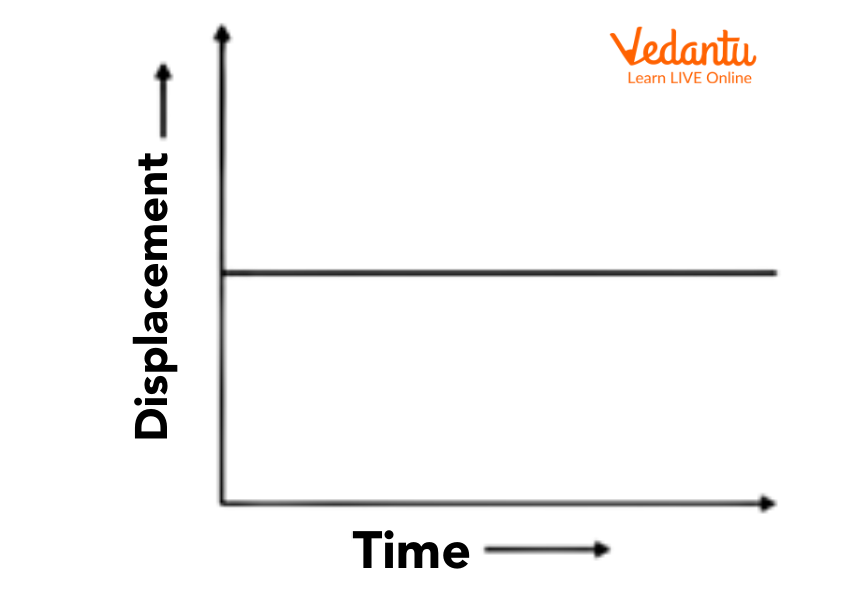Displacement- Time Graph for a Stationary Body

### 2. When a Body is in Uniform Motion:

The displacement-time graph represents the body in uniform motion when it covers equal distance in equal intervals of time with uniform velocity. The graph for uniform motion will be shown by a straight inclined line at an angle that passed through the origin.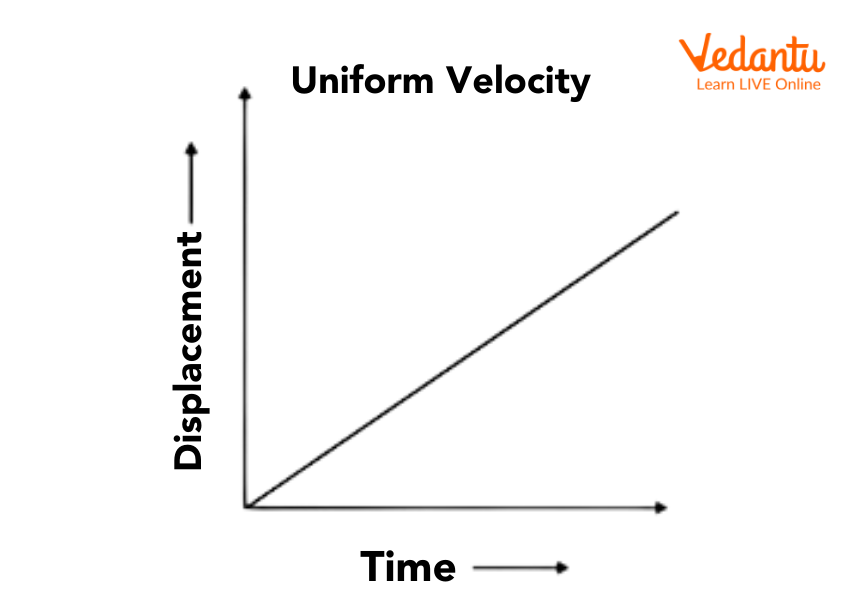Displacement-Time Graph for a Body in Uniform Motion

The velocity-time graphs also represent the uniform velocity of the body. The graphs represent it by showing a straight line that is perpendicular to the velocity axis and parallel to the time axis.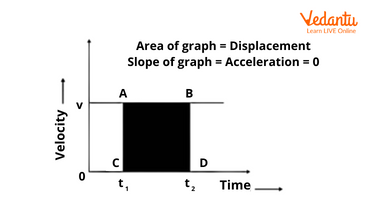Velocity -Time Graph for the Body in Uniform Motion

### 3. When the Body is in Non-Uniform Motion:

When a body is moving with non-uniform motion, it means that the change in velocity with respect to time is not constant. It clearly explains that the acceleration of the body changes with respect to time. The graph will be shown by a curved line in the velocity-time graphs.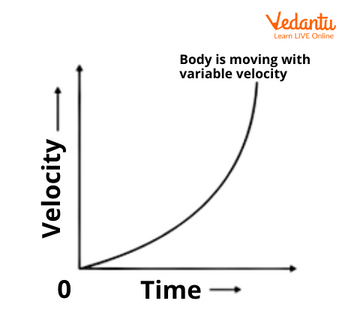Velocity-Time Graph for a Body in Non-Uniform Motion

### 4. When the Body is in Uniform Accelerated Motion:

When a body is in uniform accelerated motion, the acceleration of the body changes uniformly with respect to time. This graph is shown in the acceleration time graph by a straight line that is inclined at an angle and passes through the origin. The slope of acceleration time gives a quantity called Jerk.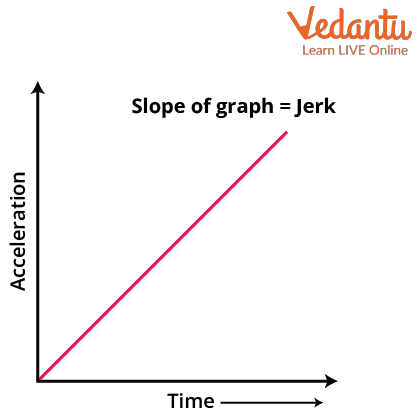Acceleration-Time Graph for a Body is in Uniformly Accelerated Motion

## Equations of Motion by Graphical Method

In kinematics, there are three equations of motion of the body. Every equation is defined with the parameters of the motion which are given as distance, displacement, velocity, acceleration and time. There are a few symbols for the parameter of the equation of motion given below:

v= Final velocity

u= Initial velocity

a= Acceleration

t= Time

s= Displacement

### 1. The First Equation of Motion

In the first equation of motion, there is a relationship established between the velocities and acceleration of the body where displacement is not given in the problem. This relationship is known as the velocity-time relation. The first equation of motion is given as:

$v=u+at$

The kinematic diagram and graph for this equation of motion is shown below: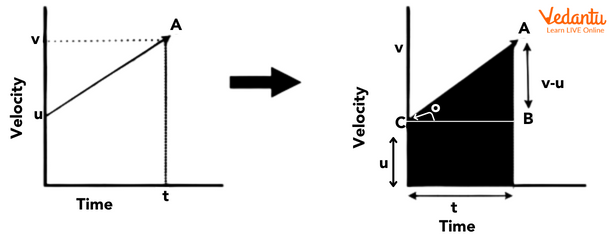Graph for the First Equation of Motion

### 2. The Second Equation of Motion:

In the second equation of motion, there is a relationship established between the velocity and time, where initial and final velocity with the displacement is made by the body. The second equation of motion is given as:

$s=ut+\dfrac{1}{2}at^2$

The kinematic diagram and graph for this equation of motion is shown below: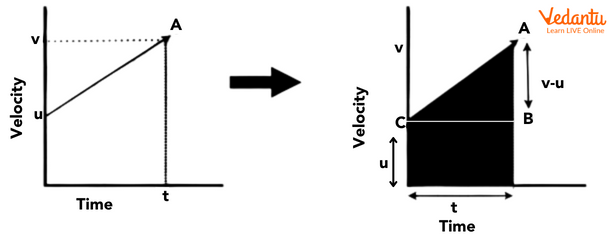Graph for Second Equation of Motion

### 3. Third Equation of Motion:

In the third equation of motion, there is a relationship established between speed and time. The third equation of motion is given as:

$v^2-u^2=2as$

This kinematic diagram and graph for this equation of motion is shown below: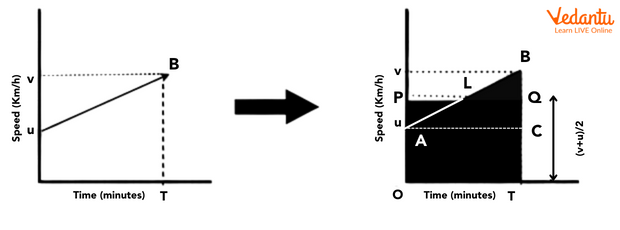Graph for the Third Equation of Motion

## Conclusion

This article concludes with the conceptual information regarding the concept of motion of the body, defines kinematics and the equation of motion. The graphical analysis of the motion of the body has been shown in an elaborate manner. The article discusses the kinematics graphs of the kinematic equation of motion.

In the given article, various types of motion have been shared along with their respective graphs.  This article also consists of the kinematic equation of motion through the graphical method. This article also showed the types of motion of the body in stationary, uniform and non-uniform motion, uniformly accelerated motion graphs and along with their graph equations i.e., kinematic equations.

## FAQs on Graphical Analysis of Kinematics - JEE Important Topic

1. What is the meaning of kinematics? Give real-life examples of kinematics.

Kinematics is the branch of mechanics which is used for the study of the motion of the body, without considering the cause of the motion. This analysis required various parameters for identifying the motion of particles. Those parameters are given as displacement, velocity and acceleration. Some of the common real-life examples of kinematics are the motion of particles: a car moving on a straight road, a man walking on the street, a train moving from one station to another etc.

2. What is the importance of kinematics for the JEE Main exam?

Mechanics is one of the important topics for both the . From kinematics, questions based on graphical representation and equations of motion are frequently asked in JEE exams. Proper revision of concepts and formulas can help you secure better marks in exams. In 2022, a total 2 questions were from the kinematics section in mechanics making the weightage of about 6.6% from total marks. This topic also forms a base for several numerical and multiple-choice questions for mechanics.